# ML Aggarwal Class 6 Solutions for ICSE Maths Chapter 1 Knowing Our Numbers Check Your Progress

## ML Aggarwal Class 6 Solutions for ICSE Maths Chapter 1 Knowing Our Numbers Check Your Progress

Question 1.
Write the numeral for each of the following numbers and insert commas correctly:
(i) Six crore nine lakh forty seven.
(ii) One hundred four million seven hundred twenty two thousand three hundred ninety four.
Solution:Question 2.
Insert commas suitably and write the number 30189301 in words in Indian and International system of numeration.
Solution: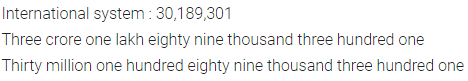Question 3.
Find the difference between the place value and the face value of the digit 6 in the number 72601.
Solution: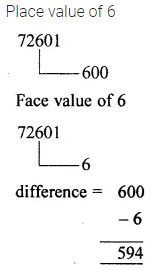Question 4.
Write all possible two-digit number using the digits 4 and 0. repetition of digits is allowed.
Solution:Question 5.
Write all possible natural numbers using the digits 7, 0, 6. Repetition of digits is not allowed.
Solution:Question 6.
Arrange the following numbers in ascending order:
3706, 58019, 3760, 59801, 560023
Solution:Question 7.
Write the greatest six-digit number using four different digits.
Solution:Question 8.
Write the smallest eight-digit number using four different digits.
Solution: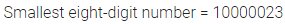Question 9.
Find the difference between the greatest and the smallest 4-digit numbers formed by the digits 0, 3, 6, 9.
Solution: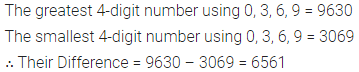Question 10.
Find the sum of the four-digit greatest number and the five-digit smallest number, each number having three different digits.
Solution: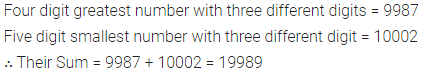Question 11.
Write the greatest and the smallest four-digit numbers using four different digits with the conditions as given:
(i) Digit 3 always at hundred’s place.
(ii) Digit 0 always at ten’s place.
Solution: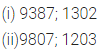Question 12.
A mobile number consists of ten digits. First four digits are 9, 9, 7 and 9. Make the smallest mobile number by using only one digit twice from the digits 8, 3, 5, 0, 6.
Solution:Question 13.
Two stitch a uniform, 1 m 75 cm cloth is needed. Out of 153 m cloth, how many uniforms can be stitched and how much cloth will remain?
Solution: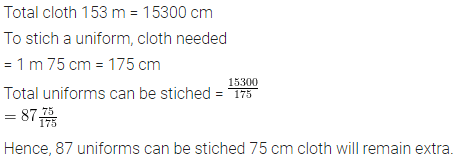Question 14.
Medicine is packed in boxes, each weighing 4 kg 500 g. How many such boxes can be loaded in a van which cannot carry beyond 800 kg?
Solution:Question 15.
Estimate : 6554 – 677 by estimating the numbers to their nearest
(i) thousands
(ii) hundreds
(iii) greatest places
Also point out the most reasonable estimate.
Solution: